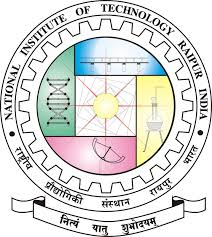Crystal Clear
Tag(s):

## Medium

Problem
Editorial
Analytics

After a tiring day of manufacturing high quality meth, Jesse and Walter were busy packing the supplies in the boxes. Considering it a boring task, Walter asked Jesse to automate the packaging process. As Walter considers automation can be faulty sometimes, he designs a simple algorithm to test the quality of the packaging received at end.

The algorithm goes as follows:

• Consider pairs of indexes [Li,Ri], where every pair represents a contiguous segment of boxes starting and index Li and ending at index Ri. The segments can overlap.
• Walter has assigned some integeral value Ai to each of these segments for $1<=i<=M,$ corresponding to the quality of that segment.
• Initially,Quality=0. For every segment i, if there is atleast one box containing blue meth in the given segment, then A is added to total quality.  (Note: Acan be negative too).

Jesse has asked you to help him. He wants to place the boxes containing high quality blue meths in such a way, that the total Quality for the batch is Maximised. Help him find this maximum value.

Input

The first line contains two integers N and M (1 ≤ N,M ≤ 2*105) — corresponding to the number of boxes in the batch, and total number of segments given by Walter.

Next lines contains 3 space seperated integers, Li  ,Ri , A each.

•  $1 ≤ L i ≤ R i ≤ N$
• $| A i | ≤ 10^ 9$

Output

Print a single integer, the Maximum value of total Quality that can be achieved.

SAMPLE INPUT
6 8
5 5 3
1 1 10
1 6 -8
3 6 5
3 4 9
5 5 -2
1 3 -6
4 6 -7
SAMPLE OUTPUT
10
Explanation

In the given sample, Optimum solution is to place blue meths in boxes numbered 1 and 3 and normal meths in rest all of the batch. This gives us 2nd,3rd,4th,5th,7th  segments to be considered for total quality.

Thus total quality is: 10+ (-8) + 5 + 9 + (-6) =10, which comes from adding 2nd,3rd,4th,5th,7th  segments values to total quality.

You can verify that this is the maximum possible quality which can be attained.

Time Limit: 3.0 sec(s) for each input file.
Memory Limit: 256 MB
Source Limit: 1024 KB
Marking Scheme: Marks are awarded when all the testcases pass.
Allowed Languages: Bash, C, C++, C++14, Clojure, C#, D, Erlang, F#, Go, Groovy, Haskell, Java, Java 8, JavaScript(Rhino), JavaScript(Node.js), TypeScript, Julia, Kotlin, Lisp, Lisp (SBCL), Lua, Objective-C, OCaml, Octave, Pascal, Perl, PHP, Python, Python 3, R(RScript), Racket, Ruby, Rust, Scala, Swift, Swift-4.1, Visual Basic

## CODE EDITOR

Initializing Code Editor...

## This Problem was Asked inChallenge Name

Breaking Code 3.0

OTHER PROBLEMS OF THIS CHALLENGE
• Algorithms > Graphs

?